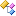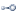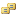# AGI.Foundation.Radar Namespace

Contains types that perform radar system performance analysis.Classes
ClassDescriptionConstantEfficiencyFixedNumberOfPulsesWaveformIntegrator
A fixed number of pulses integrator which computes the integrated signal-to-noise ratio based on a constant efficiency. The integrator adjusts the fixed number of pulses by the constant efficiency and then uses the adjusted number of pulses in order to compute the integrated signal-to-noise ratio using the single pulse signal-to-noise ratio.ConstantEfficiencyGoalSignalToNoiseRatioWaveformIntegrator
A goal signal-to-noise ratio integrator which computes the integrated number of pulses and integrated signal-to-noise ratio based on a constant efficiency. The integrator multiplies the single pulse signal-to-noise ratio by the constant efficiency value in order to compute the integrated number of pulses and signal-to-noise ratio.DwellTimeScalar
A SingleTargetRadarLinkScalar which represents the radar waveform dwell time, in seconds. The dwell time is defined as the total integration time plus the two way RF propagation time of the radar waveform.EffectiveIsotropicRadiatedPowerScalar
A SingleTargetRadarLinkScalar which represents the effective isotropic radiated power (EIRP), in Watts, of the radar transmitter. EIRP is computed by multiplying the transmitted signal power by the transmit antenna gain in the direction of the target.FixedNumberOfPulsesWaveformIntegrator
A radar waveform integrator which will integrate a fixed number of pulses in the waveform.GoalSignalToNoiseRatioWaveformIntegrator
A radar waveform integrator which will integrate as many pulses as necessary in order to reach a goal signal-to-noise ratio.IntegratedPulseCountScalar
A SingleTargetRadarLinkScalar which represents the number of pulses integrated in the received waveform.InterferenceSignalIdentifier
An identifier added to a radar interference signal. This identifier is intended to wrap an existing instance of SignalIdentifier, which is the identifier of the interfering transmit source.MitchellWalkerProbabilityOfDetectionScalar
A SingleTargetRadarLinkScalar which represents the Mitchell-Walker probability of detection model. The probability of detection (PDet) is a function of the signal-to-noise ratio (single pulse or integrated, with or without interference effects), the number of pulses integrated, the probability of false alarm, and the radar cross section (RCS) fluctuation type (SwerlingTargetModel). If enabled, the probability of detection for a constant false alarm rate (CFAR) can be evaluated based on the number of reference cells. A CFAR receiver will adjust the detection threshold based on the noise in reference 'cells' around the cell being examined for the presence of a target.MonostaticAmbiguousTargetRangeScalar
A SingleTargetRadarLinkScalar which represents the radar ambiguous target range. The range is ambiguous where the true target range is greater than the computed unambiguous range value. The ambiguous range is computed by using the equation r = t % u, where "r" is the ambiguous range, "t" is the true range and "u" is the unambiguous range computed from the waveform pulse repetition frequency. This scalar assumes a monostatic radar geometry, meaning that the radar transmitter and radar receiver are co-located.MonostaticAmbiguousTargetVelocityScalar
A SingleTargetRadarLinkScalar which represents the radar ambiguous target velocity. The velocity is ambiguous where the true target velocity is greater than the computed unambiguous velocity value. The ambiguous velocity is computed by using the equation v = t % u, where "v" is the target closure (negative of the range rate), "t" is the true velocity and "u" is the unambiguous velocity computed from the waveform pulse repetition frequency. This scalar assumes a monostatic radar geometry, meaning that the radar transmitter and radar receiver are co-located.PerfectFixedNumberOfPulsesWaveformIntegrator
A fixed number of pulses integrator which computes the integrated signal-to-noise ratio based on perfect integration. The integrator uses the single pulse signal-to-noise ratio multiplied by the number of pulses in order to compute the integrated signal-to-noise ratio.PerfectGoalSignalToNoiseRatioWaveformIntegrator
A goal signal-to-noise ratio integrator which computes the integrated number of pulses and integrated signal-to-noise ratio based on perfect integration. The integrator uses the single pulse signal-to-noise ratio in order to compute the integrated number of pulses and signal-to-noise ratio.ProcessedRadarWaveform
An immutable data container which represents the radar waveform after being processed by the radar receiver signal processing.ProcessedRadarWaveformOutputExtension
An extension which identifies the output of a radar receiver's waveform processing subsystem.PulseIntegrationTimeScalar
A SingleTargetRadarLinkScalar which represents the time, in seconds, it takes for the radar receiver to integrate the number of pulses in the received waveform.RadarAntennaSignalProcessor
Represents a receiving radio frequency (RF) antenna. By default, an instance of ParabolicGainPattern is used as the gain pattern and signals not within the band MinimumRfFrequency to MaximumRfFrequency are rejected from the output.RadarReceivingAntennaExtension
An ObjectExtension which represents a radar receiving antenna.RadarTransmittingAntennaExtension
An ObjectExtension which represents a radar transmitting antenna.RadarWaveformProcessor
Represents a radar signal processor that produces a ProcessedRadarWaveform instance from the Signal scattered from the target as well as any interference signals.ReceiveAntennaGainScalar
A SingleTargetRadarLinkScalar which represents the radar receiver antenna gain, as a unitless ratio, in the direction of the target object.ReceiveAntennaOutputInterferencePowerScalar
A SingleTargetRadarLinkScalar which represents the interference power level at the output of the receive radar antenna. This value is computed by multiplying the received isotropic power by the antenna gain in the direction of the target object.ReceiveAntennaOutputPowerScalar
A SingleTargetRadarLinkScalar which represents the power level at the output of the receive radar antenna. This value is computed by multiplying the received isotropic power by the antenna gain in the direction of the target object.SignalSwerlingTargetModel
A data object to be added to a Signal which represents the radar cross section Swerling target model.SignalToNoiseRatioScalar
A SingleTargetRadarLinkScalar which represents the signal-to-noise (unitless ratio), either single pulse or integrated and with or without interference effects, at the output of the radar receiver.SingleTargetRadarLinkScalar
A scalar which represents a two way radar transmission from a transmitter, scattered off of a target, and back to a receiver.TargetRadarCrossSectionExtension
An ObjectExtension which represents the radar cross section of a target object.TargetRadarCrossSectionScalar
A SingleTargetRadarLinkScalar which represents the radar cross section of the target object, in meters squared.TargetScatteredPowerScalar
A SingleTargetRadarLinkScalar which represents the power, in Watts, scattered from the target object in the direction of the radar receiver.TargetToReceiverDopplerFrequencyScalar
A SingleTargetRadarLinkScalar which represents the doppler shifted frequency of the signal scattered from the target, in hertz, at the radar receiver.TargetToReceiverDopplerShiftScalar
A SingleTargetRadarLinkScalar which represents the total doppler shift of the transmitted signal, in hertz, at the radar receiver.TargetToReceiverPropagationLossScalar
A SingleTargetRadarLinkScalar which represents the total propagation loss, as a unitless ratio, between the target object and the radar receiver. The value is computed from all of the propagation loss models (e.g. FreeSpacePathLossModel) which were added to the WirelessLinkExtension of the link ExtensibleObject between the radar transmitter to the target object.TargetToReceiverReceivedIsotropicPowerScalar
A SingleTargetRadarLinkScalar which represents the received isotropic power (RIP), in Watts, for the link between the target object and radar receiver. RIP is computed by multiplying the target scattered power in the direction of the radar receiver by the total propagation loss between the target object and radar receiver.TransmitAntennaGainScalar
A SingleTargetRadarLinkScalar which represents the radar transmit antenna gain, as a unitless ratio, in the direction of the target object.TransmitterToTargetDopplerFrequencyScalar
A SingleTargetRadarLinkScalar which represents the doppler shifted transmit frequency, in hertz, at the target object.TransmitterToTargetDopplerShiftScalar
A SingleTargetRadarLinkScalar which represents the doppler shift of the transmit frequency, in hertz, at the target object.TransmitterToTargetPropagationLossScalar
A SingleTargetRadarLinkScalar which represents the total propagation loss, as a unitless ratio, between the radar transmitter and the target object. The value is computed from all of the propagation loss models (e.g. FreeSpacePathLossModel) which were added to the WirelessLinkExtension of the link ExtensibleObject between the radar transmitter to the target object.TransmitterToTargetReceivedIsotropicPowerScalar
A SingleTargetRadarLinkScalar which represents the received isotropic power (RIP), in Watts, for the link between the radar transmitter and the target object. RIP is computed by multiplying the effective isotropic power (EIRP) by the total propagation loss between the transmitter and target object.Interfaces
InterfaceDescriptionIProcessedRadarWaveformOutputService
A service which identifies the RadarWaveformProcessor representing the output of the radar receiver waveform processing subsystem.Enumerations
EnumerationDescriptionSignalToNoiseRatioComputeMode
Indicates they type of computed signal-to-noise ratio used to compute probability of detection.SwerlingTargetModel
Models, introduced by Peter Swerling, used to describe the statistical properties of a target radar cross section.